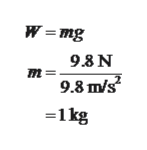5) a freely falling rock has a weight of 9.8N. The earth's mass is 6×10²⁴kg.5a) the mass of the apple is 1kg (T, F)5b) the force exterted by the earth on the rock is 9.8N (T, F)5c) the force exerted by the rock on the earth is 9.8N (T, F)5d) the acceleration of the earth is of order of 10^-23 m/s² (T, F)

Question

5) a freely falling rock has a weight of 9.8N. The earth's mass is 6×10²⁴kg.

5a) the mass of the apple is 1kg (T, F)

5b) the force exterted by the earth on the rock is 9.8N (T, F)

5c) the force exerted by the rock on the earth is 9.8N (T, F)

5d) the acceleration of the earth is of order of 10^-23 m/s² (T, F)

Step 1

5a)

The given statement is true.

The mass of the object is,help_outlineImage TranscriptioncloseW mg 9.8 N 9.8 m/s 1kg fullscreen
Step 2

5b)

The given statement is true.

For a free falling object, only gravitational force is act...

Want to see the full answer?

See Solution

Want to see this answer and more?

Our solutions are written by experts, many with advanced degrees, and available 24/7

See Solution
Tagged in

Gravitation